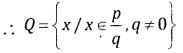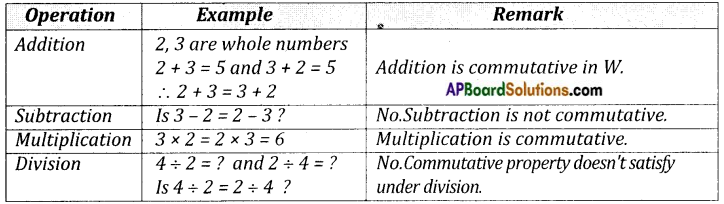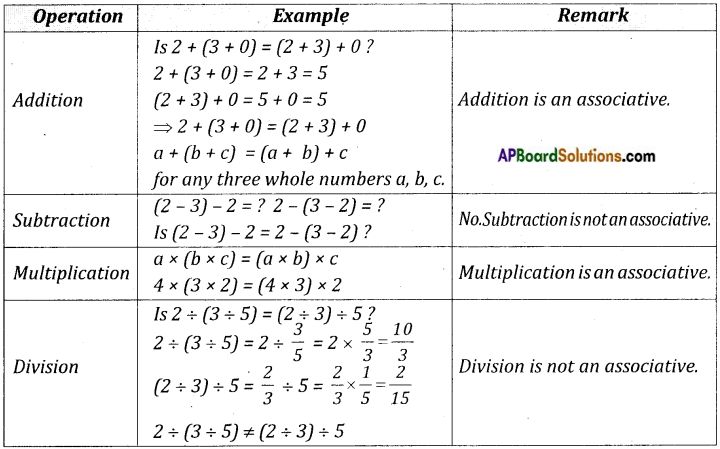# AP Board 8th Class Maths Notes Chapter 1 Rational Numbers

Students can go through AP Board 8th Class Maths Notes Chapter 1 Rational Numbers to understand and remember the concepts easily.

## AP State Board Syllabus 8th Class Maths Notes Chapter 1 Rational Numbers

→ The numbers which are expressed in the form of $$\frac{p}{q}$$ where p and q are integers and q ≠ 0, are called “Rational Numbers” which are denoted by the letter ‘Q’.→ Rational numbers are closed under the operations of addition, subtraction and multiplication.

→ Rational numbers are not closed on division.→ Whole numbers:→ Whole numbers:→ The additive inverse of a is – a. (∵ a + (-a) = 0)→ The multiplicative inverse of a is $$\frac{1}{a}$$. (∵ a × $$\frac{1}{a}$$ = 1)

→ The operations addition and multiplications are

1. Commutative for rational numbers.
2. Associative for rational numbers.

→ ‘0’ is the additive identity for rational number.

→ ‘1’ is the multiplicative identity for rational number.

→ A rational number and its additive inverse are opposite in their sign.

→ The multiplicative inverse of a rational number is its reciprocal.

→ Distributivity of rational numbers a, b and c is a(b + c) = ab + ac and a(b – c) = ab – ac.

→ Rational numbers can be represented on a number line.

→ There are infinite number of rational numbers between any two given rational numbers.→ The concept of mean help us to find rational numbers between any two rational numbers.

→ The decimal representation of rational numbers is either in the form of terminating decimal or non-terminating recurring decimals.